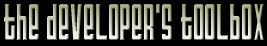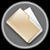This section of the archives stores flipcode's complete Developer Toolbox collection, featuring a variety of mini-articles and source code contributions from our readers.Toggle and Swap   Submitted byMany people toggle a variable between two values by using if statements. "If it's A, make it B. If it's B, make it A," But there's a nice little tip that I picked up from my Commodore 64 days that does this without the if statements.

Just subtract the current value from the sum of the two toggle values. In this example, we'll toggle between 3 and 5.

 ```int a = 3; // assign - a is 3 a = 8 - a; // toggle - a is 5 a = 8 - a; // toggle - a is 3 a = 8 - a; // toggle - a is 5 a = 8 - a; // toggle - a is 3 ```

I use this all the time. But this trick has an equally interesting sibling that I only recently discovered. (Yeah, I'm slow.)

The standard way to swap two variables is to have a temp variable. Without the temp, you'd stomp over the data of one of your variable before you're done with it.

 ```temp = a; a = b; b = temp; ```

Using a variation of the toggle trick, you can do this without using the temp variable. Just add the value of B to A before the swap to preserve it, then "toggle" the values into their new storage.

 ```int a = 3; int b = 5;a = a + b; // a = 8 b = a - b; // b = 8 - 5 = 3 a = a - b; // a = 8 - 3 = 5 ```

I don't know what an optimizer would do to this code, but I doubt it's more efficent than the swap with a temp variable since the temp variable is likely to stored in a register. I just thought it was interesting.

There's also an XOR trick which does the same thing, but it doesn't work for floats or doubles, and it doesn't work in some special cases (like A == B).

-- groundh0g

The zip file viewer built into the Developer Toolbox made use of the zlib library, as well as the zlibdll source additions.